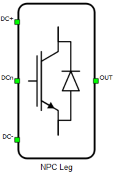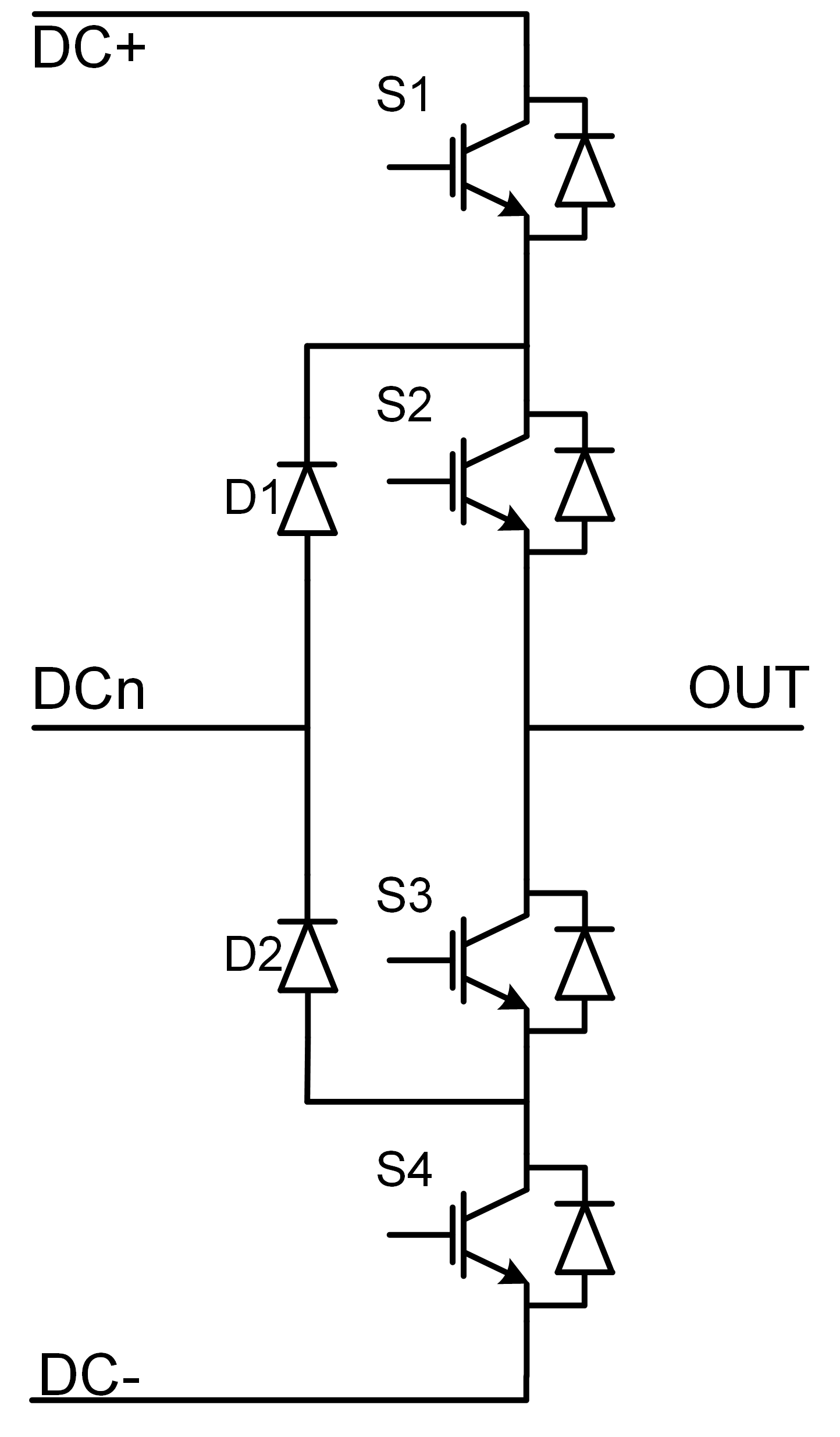# NPC Leg

This section describes the NPC Leg block in Schematic Editor

A block diagram and input parameters for a NPC Leg block is given in Table 1.

Table 1. NPC Leg block in the Schematic Editor core library
component component dialog window component parametersNPC Type Leg• Control (Digital inputs, Internal modulator, Model)
• S_1 (1..32)
• S_2 (1..32)
• S_3 (1..32)
• S_4 (1..32)
• gate_logic (active high, active low)
Weight = 1

A schematic block diagram of the inverter switching block is given in Figure 1 with corresponding switch arrangement and naming.

Digital inputs, when selected as the Control parameter, enables you to assign gate drive inputs to any of the digital input pins (from 1 to 32). For example, if S1 is assigned to 1, the digital input pin 1 will be routed to the S1 switch gate drive. In addition, the gate_logic parameter is set to either active high (i.e. high-level input voltage VIH turns on the switch), or active low (i.e. low-level input voltage VIL turns on the switch). The gate drive logic depends on your external controller design.Figure 1. A schematic block diagram of an NPC Leg block with corresponding switch naming

Internal modulator, when selected as the Control parameter, enables you to use the internal PWM modulator for driving the converter's switches instead of digital input pins. In this configuration, three additional component inputs will be present. The En input is used to enable/disable PWM modulator control, while In1 and In2 are used as referent signal inputs for the internal PWM modulator. In1 is fed to PWM channel 1 which creates gate signals for switches S1 and S3, while In2 is fed to PWM channel 2 which creates gate signals for switches S2 and S4.

Model, when selected as the Control parameter, enables you to set the IGBTs gate drive signals directly from the signal processing model. The input pin gates appears on the component and requires a vector input of four gate drive signals in the following order: [S1, S2, S3, S4]. When controlled from the model, logic is always set to active high.

DTV detection, when enabled, allows DTV detection to be signalized during simulation runtime.

Note: An interactive overview of how you can use DTV detection in your model is available as part of the HIL for Power Electronics course on HIL Academy, as well as in the video Knowledgebase.

## Timing

When Enable delays is enabled, the turn on and turn off delay of the IGBTs will be included in the simulation. More information about this feature can be found on the dedicated section switching delay.

Note: An interactive overview of how you can use switching delays in your model is available as part of the HIL for Power Electronics course on HIL Academy, as well as in the video Knowledgebase.

## Losses calculation

When the Losses calculation property is enabled, the component will calculate switching and conduction power losses for all switching elements (IGBTs and Diodes or MOSFETs). In the case of MOSFET switching elements, the diode characteristic represents the internal MOSFET body diode. Switching power losses are calculated as a function of current, voltage, and temperature using 3D lookup tables. Also, 2D input for losses is supported. When a 2D losses table is inserted, it assumes only current (I) and temperature dependence. From version 2020.3, conduction power losses can be defined as a function of current and temperature using Vt and Vd lookup tables (LUTs) (the previous option of using Vce, Rce, Vd, and Rd properties are removed). These LUTs can be 1D or 2D tables. If the LUT is a 1D table, forward voltage drop depends only on current, but if LUT is a 2D table, forward voltage drop dependence on the junction temperature is also considered. In the MOSFET case under reverse current conduction, a current sharing calculation between the MOSFET channel and the internal body diode is performed. Import options and an explanation how to correctly fill all necessary power losses parameters is described in the Importing power losses data section. All switches are distributed in three groups, and for each group, different power loss parameters can be specified (S1 and S4 are in group 1, S2 and S3 are in group 2, D1 and D2 are in group 3).

Note: An interactive overview of how you can incorporate power loss calculation in your model is available as part of the HIL for Power Electronics course on HIL Academy, as well as in the video Knowledgebase.
If the IGBT switch types are used in both losses groups, input/output terminals for power losses are vectors of ten elements (every index in the vector represent one switching element). Ordering of switching elements is given in Table 2.
Table 2. Switching elements ordering for power losses terminals in the case of IGBT switch types in both groups
Index in the vector Switching element
 S1_igbt
 S1_diode
 S2_igbt
 S2_diode
 S3_igbt
 S3_diode
 S4_igbt
 S4_diode
 D1
 D2
If the MOSFET switch types are used in both losses groups, input/output terminals for power losses are vectors of six elements (every index in the vector represent one switching element). Ordering of switching elements is given in Table 3.
Table 3. Switching elements ordering for power losses terminals in the case of MOSFET switch types in both groups
Index in the vector Switching element
 S1_mosfet
 S2_mosfet
 S3_mosfet
 S4_mosfet
 D1
 D2
If the MOSFET switch type is used for losses group 1 and IGBT switch type for losses group 2, input/output terminals for power losses are vectors of eight elements (every index in the vector represent one switching element). Ordering of switching elements is given in Table 4.
Table 4. Switching elements ordering for power losses terminals in the case of MOSFET switch type in group 1 and IGBT switch type in group 2
Index in the vector Switching element
 S1_mosfet
 S2_igbt
 S2_diode
 S3_igbt
 S3_diode
 S4_mosfet
 D1
 D2
If the IGBT switch type is used for losses group 1 and MOSFET switch type for losses group 2, input/output terminals for power losses are vectors of eight elements (every index in the vector represent one switching element). Ordering of switching elements is given in Table 5.
Table 5. Switching elements ordering for power losses terminals in the case of IGBT switch type in group 1 and MOSFET switch type in group 2
Index in the vector Switching element
 S1_igbt
 S1_diode
 S2_mosfet
 S3_mosfet
 S4_igbt
 S4_diode
 D1
 D2
• Losses groups - Switching elements group
• Current values - Switching elements current axis [A]

• Voltage values - Switching elements voltage axis [V]

• Temp values - Switching elements temperature axis [°C]

• Vce - IGBT collector emitter saturation voltage [V] - discontinued from 2020.3 release

• Rce - IGBT on state slope resistance [Ohm] - discontinued from 2020.3 release

• Vd - IGBT Diode voltage drop [V] - discontinued from 2020.3 release

• Rd - IGBT Diode slope resistance [Ohm] - discontinued from 2020.3 release

• Vt table - Switch forward voltage drop, f(I,T) [V]

• Vd table - Diode forward voltage drop, f(I,T) [V]

• Et on table - Switch switching ON losses, output energy, f(I, V, T) [J]

• Et off table - Switch switching OFF losses, output energy, f(I, V, T) [J]

• Ed off table - Diode switching OFF losses, output energy, f(I, V, T) [J]

Note: Automatic initialization of the Vt table and Vd table properties will be performed based on the discontinued Voltage and Resistance properties (Vce, Rce, Vd, Rd).

## Temperatures calculation

When the Temperatures calculation property is enabled, the component will calculate the combined power losses (P_loss) and junction temperatures (T_junctions) for all switching elements (IGBTs and diodes). Combined power losses represent the sum of the calculated switching and conduction losses transferred through an internally generated Thermal network component. The internally generated Thermal network component also calculates junction temperatures from power losses, input cases temperatures and provided thermal model parameters. For each switching group different thermal parameters can be specified. Input/output ports for temperatures calculation are vectors of ten elements and they are indexed in the same way as explained in Table 2.

Note: An interactive overview of how you can incorporate temperature calculation in your model is available as part of the HIL for Power Electronics course on HIL Academy, as well as in the video Knowledgebase.
• Thermal networks type- Defines type of internal thermal network
• Rth switch - List of thermal resistance for the IGBT switch
• Tth switch / Cth switch - List of thermal time constants or thermal capacitances for the IGBT switch
• Rth diode - List of thermal resistance for diode
• Tth diode / Cth diode - List of thermal time constants or thermal capacitances for diode
• Calculations execution rate - Execution rate in [s] for the losses and temperatures calculation logic

## PESB Optimization

The PESB Optimization option is available in certain converter models. When PESB Optimization is enabled, all converter's short circuit state space modes will be merged and treated as the same state space mode. For example, if one converter leg within the three phase converter is short circuited and PESB Optimization is enabled, all of the legs within the three phase converter will also be short circuited. This simplification for short circuit modeling can save a significant amount of matrix memory.

## Digital Alias

If a converter is controlled by digital inputs, an alias for every digital input used by the converter will be created. Digital input aliases will be available under the Digital inputs list alongside existing Digital input signals. The alias will be shown as Converter_name.Switch_name, where Converter_name is name of the converter component and Switch_name is name of the controllable switch in the converter.